# How to Calculate Percentage in ExcelClaudia Buckley#### Join the Excel conversation on Slack

Ask a question or join the conversation for all things Excel on our Slack channel.

If you do lots of work in Excel, you probably do lots of work with numbers. And if you do lots of work with numbers, then you’ll need to calculate percentages quite often.

In this tutorial, we’ll cover how to calculate percentage in Excel. Let’s start off with the basics.

## Standard mathematical operators in Excel

It helps to know how the four basic mathematical operations are represented in Excel.

Operation

Excel Character

+

Subtraction

-

Multiplication

*

Division

/

Now let’s get busy.

Use this free Excel percentage file to practice along with the tutorial.

## How to format values as percentages

You may have seen a percentage icon in the Number command group on the Home tab. This icon changes number formats to percentages.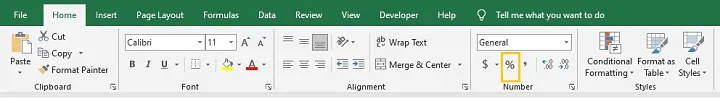It does this by multiplying the value by 100 and adding a percent sign. This works great if the value you have is a decimal. But of course, if you had a whole number, then clicking the percent icon will multiply your value by 100 and will probably not be the result you want.

For example, in the image below, the active cell, A1, contains the number 5 and carries a general format.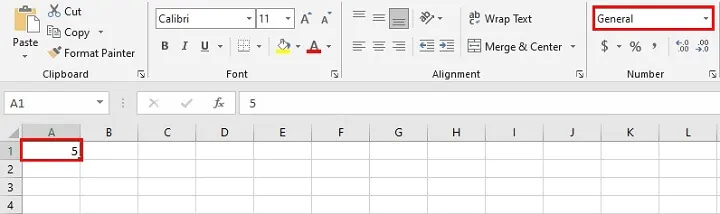Clicking the percent icon will change the value 5 to 500% because Excel has multiplied the number by 100.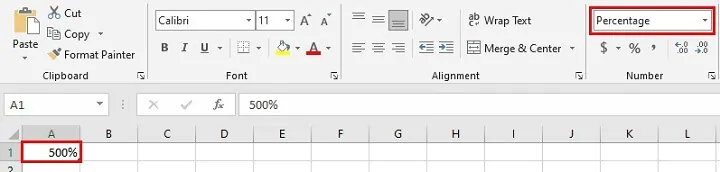On the other hand, if you format a cell as Percentage first, then add numeric values, Excel will automatically append the percent sign to the number. So the 25 was typed into cell A3 below, which was previously formatted as Percentage. Excel displays this number as 25%.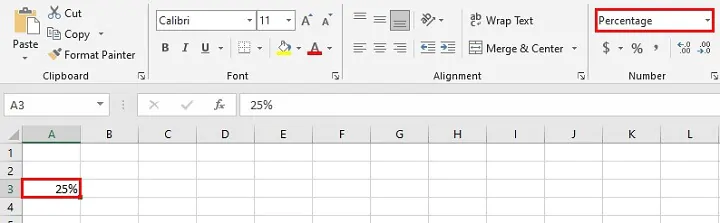However, if we change cell A3 to General format, the value is displayed as 0.25.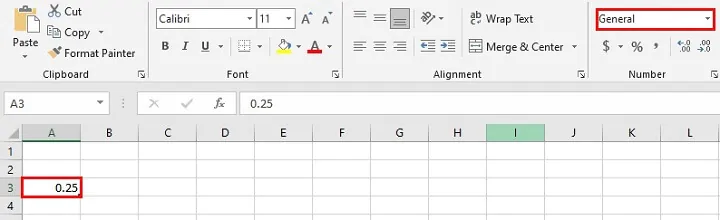Here’s a hot tip: This teaches us that number formats don’t change the value of a number. They only change the number’s appearance.

Let’s get down to how we actually calculate the percentage of something.

## Basic Excel percentage formula

One thing to note is that there isn’t a function to calculate percentages. The mathematical formula for percentages is that there is a value being expressed as the fraction of a whole, then multiplied by 100. If you would like to calculate the percentage of a total, then you just need to do that same thing in Excel.

Usually, when we want to calculate percentages and the denominator is 10 or 100, that’s fairly simple and is often calculated mentally. But whenever the denominator is not a factor of 100, it gets a little trickier to do without a bit of help. So if a student scores 13 out of 17 in a quiz, what percent did they get on their quiz? We can create a simple Excel formula for that.

Excel formulas start with an equal sign, and we use a forward slash to express fractions.

So we would enter:

``=13/17``

A value of 0.76 will be displayed. Then if we change the cell format to Percentage (remember - this multiplies the value by 100), we’ll get 76% displayed in the cell.

Alternatively, we could have formatted the cell as Percentage first, then typed =13/17. This would display 76% as the result.

If we have several of these values to calculate, we can perform this calculation even easier by placing the denominator in a single cell and referring to it as a fixed value.

``=B3/\$B\$1``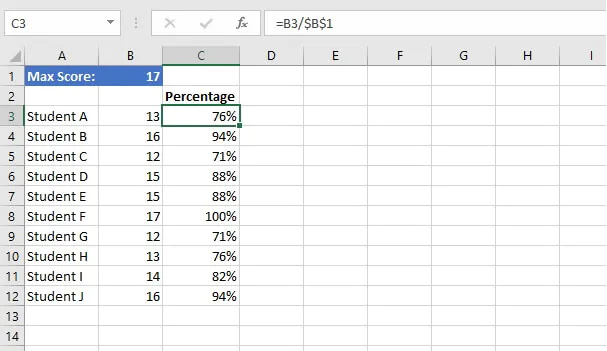Placing dollar signs before the column name and the row number when referring to cell B1 ensures that when this formula is copied to any other cell, the reference does not shift. This is known as an absolute reference

Notice that B3 is not entered as a fixed reference. Therefore, when the formula is copied to the cells below, B3 is changed to B4 for the formula in C4, to B5 for the formula in C5, and so on.

## Increase a number by a percentage

Here is a rather common situation. What if you know the percentage increase you want, and just need help calculating the result? That’s quite easy too.

Typically, we know how much profit we’d like to make on goods being sold. Let’s say we want to mark up our items for sale by 47%. This means that our goods will be sold for the original cost plus 47% of their original cost. So the selling price will be 147% of the original figure.

``=B2 * (1+47%)``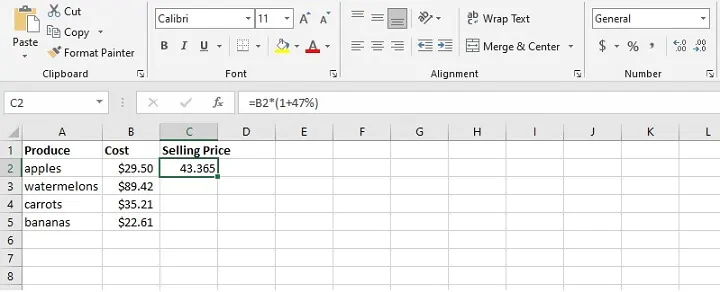Writing the formula this way eliminates the manual steps of finding out 47% of the cost, and then adding it to the original figure. By telling Excel to add 1 to 47%, then multiply it by the original we’re asking, “What is 147% of the cost?”

Notice that the result defaults to the General number format. Since we want the answer displayed as dollars, we can either click the dollar sign icon, or the Number format dropdown and select Currency, where there are other currency types to choose from.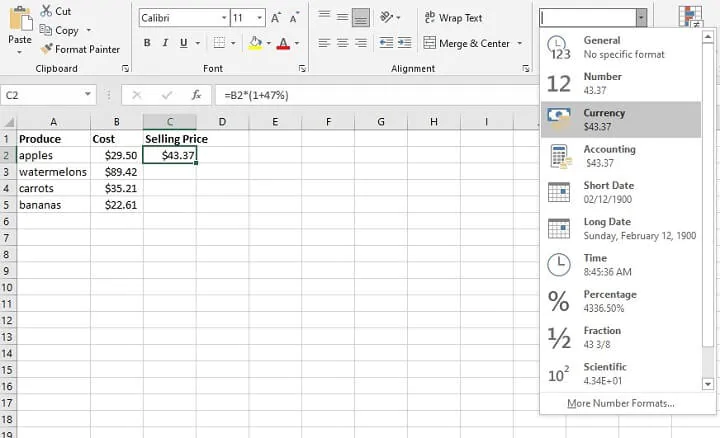We can now copy C2 to the remaining rows, and both the formula and cell format will be copied.

## Decrease a number by a percentage

Decreasing something by a percentage works in a similar way, but instead of adding the percentage, we would subtract it from the whole.

When offering a 33% sale, we are really selling the goods for 67% of the original price (i.e., 100% - 33%). To calculate this in one step in Excel, we would use the formula:

``=B2 *(1-33%)``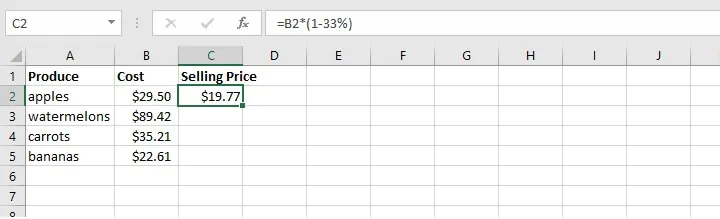We can also change the number of decimal places by clicking on the Increase or Decrease decimal icons.

## Calculate percentage change between numbers

What if you wanted to answer the reverse question. Let’s say you have the “before” and “after” figures, and wanted to know what the percentage increase or decrease was?

In the image below, February showed an increase for some cities and a decrease for others. We want Column D to show February’s change as a percentage increase or decrease over January.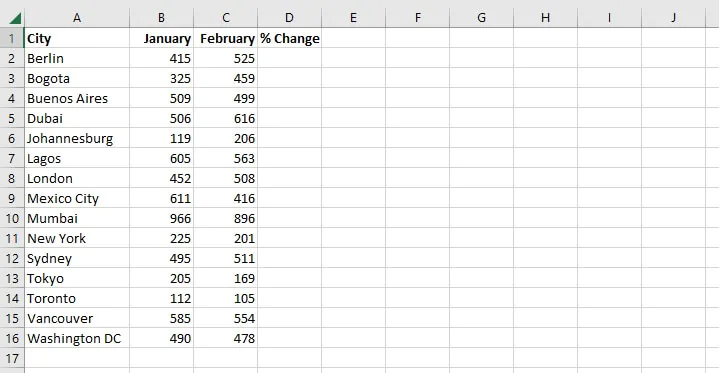So first, we need to determine what that change is by subtracting the old figure from the new figure. In the first row, that would mean:

``=C2-B2``

The result is 110. This value should now be expressed as an increase over the original figure. We can return to cell D2 and place the formula within parentheses, then instruct Excel to divide the result by January’s figure:

``=(C2-B2)/B2``

Cells default to the General number format, so the value that is returned will be shown as a decimal. We already know that if we click the percent icon, then the increase or decrease will be multiplied by 100 and shown as a percentage.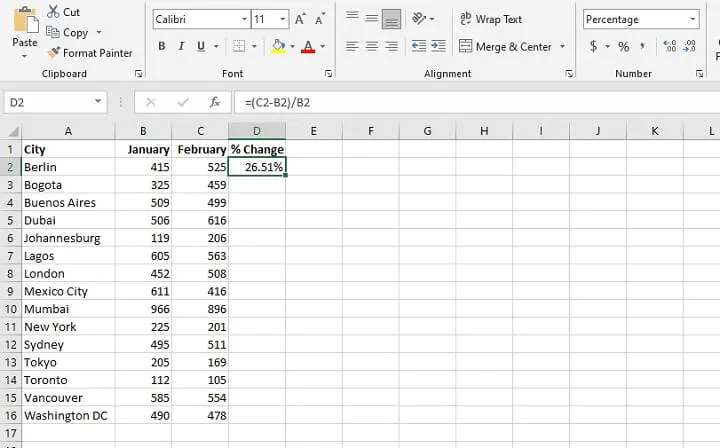Here’s another tip: You can increase or decrease the number of decimal places displayed in a cell by clicking the Increase Decimal or Decrease Decimal icon from the Number command group on the Home tab.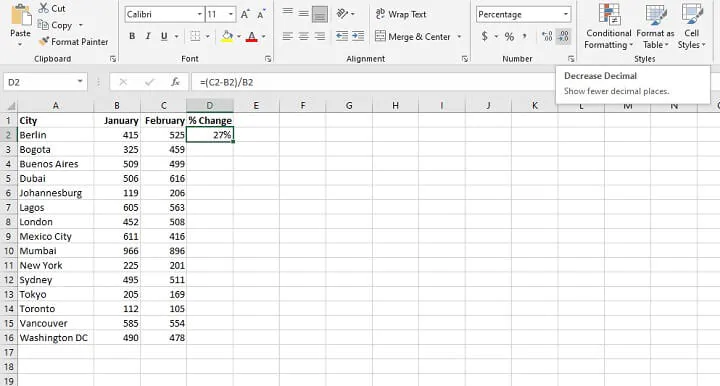We can now copy D2 to the remaining rows, and both the formula and cell format will be copied.

### Create a custom number format

We may want to customize the appearance of numbers, maybe by making the percentage decreases (negative values) stand out in red.

1. Highlight the cells to which you want to apply the customized format.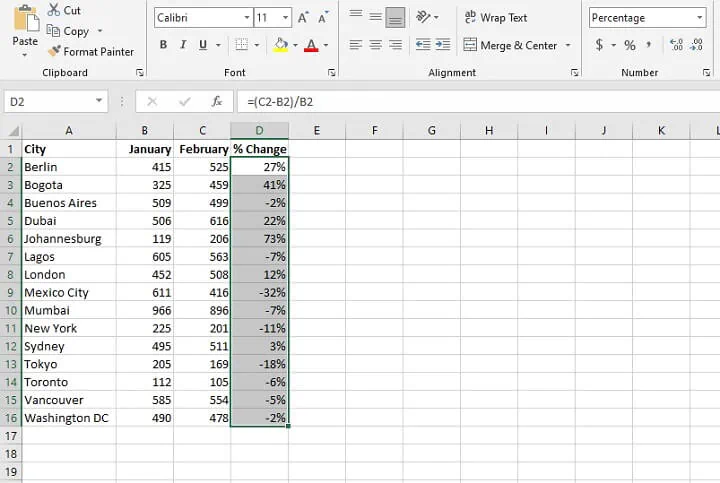1. Click on the More Number Formats option from the dropdown in the Number command group.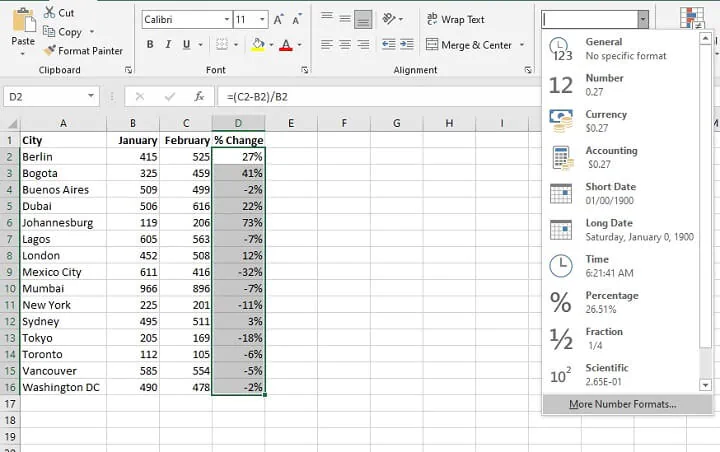1. From the Number tab, select the Custom category, and use one of the existing codes as a starting point to create and save a customized format. If you want one that places negative values in red, look for a format that has [Red] in the second position, which shows how negative numbers will appear. You may also add or remove parentheses for negative values. Since column D is made up of cells calculating percentages, place a percent sign before the semicolon, and a percent sign at the end of the format. Your customized format will look something like this:

#,##0%;[Red]#,##0%

This means that positive numbers will be rounded to the nearest whole number and carry a percent sign. Negative numbers will be displayed in red, rounded to the nearest whole number, and carry a percent sign. In both cases, values of 1000 or higher will have a comma separator.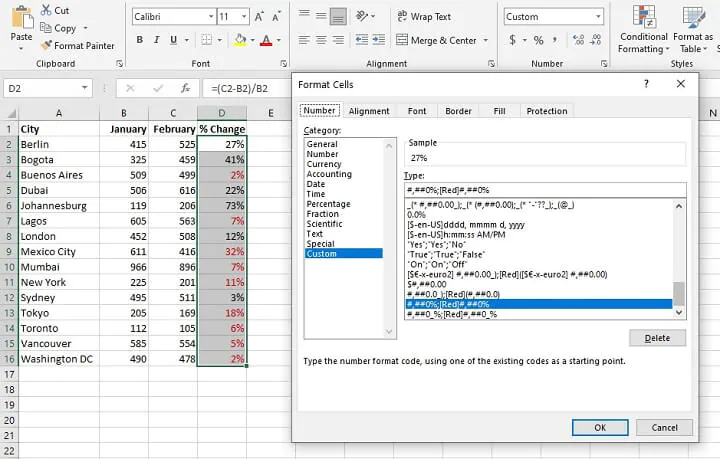Once you click OK, the new format will be applied to the selection. Of course, you could have also adjusted the number of decimal places from the Increase or Decrease Decimal icons in the Number command group.

That’s how to calculate percentage in Excel. Now you can kiss manual calculation of percentages goodbye with Excel’s easy-to-use percentages.

Learn how to use Excel like a pro with our Basic and Advanced course. Or you can start with the free Excel in an Hour course to cover some basics in Excel.

Start learning formulas, functions, and time-saving hacks today with this free course!#### Join the Excel conversation on Slack

Ask a question or join the conversation for all things Excel on our Slack channel.Claudia Buckley

Claudia is a project manager and business skills instructor at GoSkills. In her spare time, she reads mystery novels and does genealogy research.